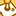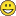# QlikView App Dev

Discussion Board for collaboration related to QlikView App Development.

Announcements
Welcome to Qlik Community! Check out our new navigation! FIND OUT MORE
cancel
Showing results for
Did you mean:Contributor II

## Qlik Sense - IF inside AVG or SUM

Hi everyone, today i tried to calculate an AVG for a metric like this:

```Avg(DISTINCT [Price])
```

But after that the user send me change, "We need calculate the price based in type of client, if client is type B the metric is Price * 1.21 and if the client isn´t B the metric is Price directly"

If i write separedly:

```Avg({<[Client_Type]={'B'}>}DISTINCT [Price]*1.21)
```

```Avg({<[Client_Type]=-{'B'}>}DISTINCT [Price])
```

The expressions working correctly, but i cant find a way to calculate this average completly.

I tried with:

```(Avg({<[Client_Type]={'B'}>}DISTINCT [Price]*1.21)+Avg({<[Client_Type]=-{'B'}>}DISTINCT [Price]))/2
```

But this expression shows a wrong value.

Is possible put a condicional calculation based in a dimension , inside a AVG or SUM  to show in a metric ?

Thanks in advanced1 Solution

Accepted SolutionsMVP

This is one option

Avg(DISTINCT If(Client_Type = 'B', [Price] * 1.21, [Price]))

2 RepliesMVP

This is one option

Avg(DISTINCT If(Client_Type = 'B', [Price] * 1.21, [Price]))Contributor II
Author

Really Thanks